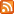# Re: Untitled

From 李清璇, 3 Years ago, written in C, viewed 648 times. This paste is a reply to Untitled from 李清璇 - view diff
Embed1. #include<stdio.h>
2. #include<stdlib.h>
3. #include<string.h>
4. struct
5. {
6.     char name;
7.     int m;
8. } stu ;
9. int main()
10. {
11.
12.     int n,i,x,y,l=0,p,j,t;
13.     char q;
14.     scanf("%d",&n);
15.     for(i=0; i<n; i++)
16.     {
17.
18.         scanf("%s %d",&stu[i].name,stu[i].m);
19.
20.     }
21.     scanf("%d %d",&x,&y);
22.     for(i=0;i<n-1;i++)
23.     {
24.         for(j=i+1;j<n;j++)
25.         {
26.              if(stu[i].m>stu[j].m)
27.              {
28.                  t=stu[i].m;
29.                  stu[i].m=stu[j].m;
30.                  stu[j].m=t;
31.                 strcpy(q,stu[i].name);
32.                  strcpy(stu[i].name,stu[j].name);
33.                  strcpy(stu[j].name,q);
34.              }
35.         }
36.     }
37.     for(i=0; i<n; i++)
38.     {
39.         if(stu[i].m<x||stu[i].m>y)
40.             l++;
41.     }
42.     if(l==n) printf("No\n");
43.     for(i=0; i<n; i++)
44.     {
45.
46.        if(stu[i].m>=x&&stu[i].m<=y)
47.             printf("%s %d\n",stu[i].name,stu[i].m);
48.
49.     }
50.
51.     return 0;
52.
53. }
54.

# Replies to Re: UntitledTitle Name Language When
Re: Re: Untitled 李清璇 c 3 Years ago.